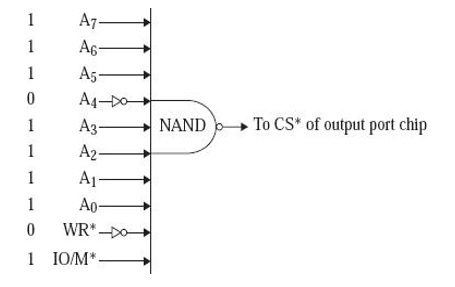# I/O-mapped I/O in 8085 Microprocessor

Generally, a processor like 8085, to address one I/O port by sending out 8-bit port address and IO/M* = 1. For example, let us say, the chip select pin of an I/O port chip is activated when 8-bit address = F0H, IO/M* = 1, and RD* = 0. This is shown in the following fig.Such I/O ports, which are addressed by the processor by sending out IO/M* as logic 1 are called I/O-mapped I/O ports.

An Input Output port is generally addressed by 8085 Processor by releasing the port address of 8-bit and IO/M* = 1. An example to be cited as the chip select pin of an Input Output port chip gets activated when the bit address of 8 bit is equal to F0H, and IO/M* is equal to 1 and RD* is equal to 0. We have shown the entire pin diagram in the following fig.

In 8085 Instruction set, there are two instructions in 8085 for communication with I/O ports. They are the IN and OUT instructions. The IN or OUT instruction mnemonic should be followed by an 8-bit port address. Thus we can have 2 8 = 256 input ports and 256 output ports are possible in 8085-based microcomputer. IN and OUT both are 2-Bytes instructions.

Mnemonics, OperandOpcode (in HEX)Bytes